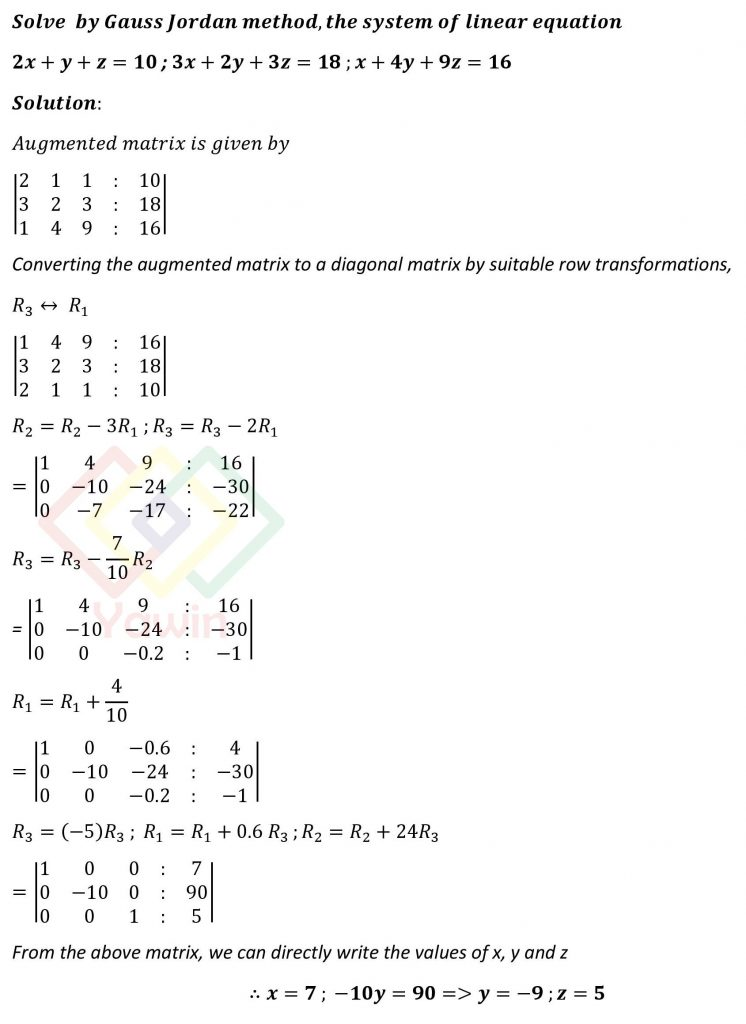Solve by Gauss Jordan method the system of linear equation 2x+y+z=10 ; 3x+2y+3z=18 ; x+4y+9z=16

## Question## Question

Solve by Gauss Jordan method the system of linear equation 2x+y+z=10 ; 3x+2y+3z=18 ; x+4y+9z=16